Disclosure: This post may contain affiliate links, meaning when you click the links and make a purchase, we receive a commission.

# INDEX MATCH vs VLOOKUP vs DGET Excel Functions (Comparison)

Microsoft Excel provides efficient functions that allow one to search for and lookup data. Of the lookup functions – we will compare INDEX MATCH vs VLOOKUP vs DGET Excel functions. DGET is not known to all. It is a database function and very useful.

The syntax for the VLOOKUP Function is the following:

=VLOOKUP (lookup_value, table_array, col_index_num, [range_lookup])

• Where lookup_value is the value you want the function to use in order to find the matching output value,
• table_array is the range that contains all the columns of interest,
• the col_index_num specifies the number of the column containing the output value that we want to look up,
• [range_lookup] is a true or false value – where TRUE denotes an approximate match and FALSE is an EXACT match.

The syntax for the INDEX and MATCH combined formula for lookups is the following:

=INDEX (range, MATCH (lookup_value, lookup_range, match_type))

• where range is the destination range that contains the value one wants to lookup, and the subsequent match function in this combination formula tells the index function the position of that value in order to retrieve it.

The syntax for the DGET function is the following:

=DGET (database, field, criteria)

• where database is the entire data set and the first row contains the labels or column headings, field indicates the column that is used in the function, and criteria is the group of cells that contains the conditions that one wants to specify.

Read More: Index Function Excel [Examples, Make Dynamic Range, INDEX MATCH]

Each lookup function has its advantages and associated disadvantages. So, let’s get started with a simple example to illustrate those advantages and disadvantages.

## Introduction

The United States Tennis Association has a rankings system, called the National Tennis Ratings Program (NTRP) which designates NTRP ratings based on the tennis player’s skill level. For the Adult NTRP Ratings, skill level is designated by looking at the results of the player at hand, in USTA League and/or USTA NTRP adult tournaments. The scale starts at 2 and increases in increments of 0.5 all the way up to 7.

A hypothetical tennis coach is interested in retrieving the USTA rankings of certain players at his clinics, in order to pitch equally ranked players against each other in future matches. The source data is shown below: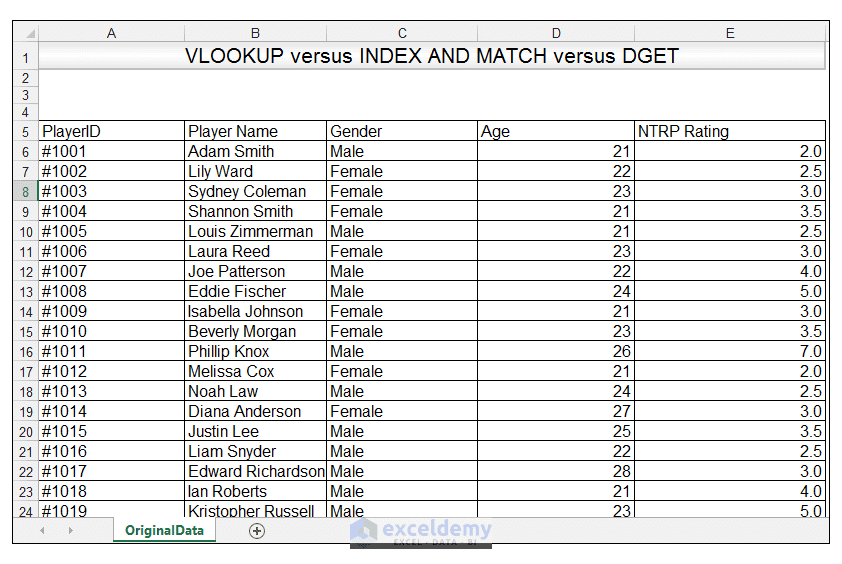## VLOOKUP

1) For our example, we want to enter a player’s name and then find their NTRP rating, using the VLOOKUP Function.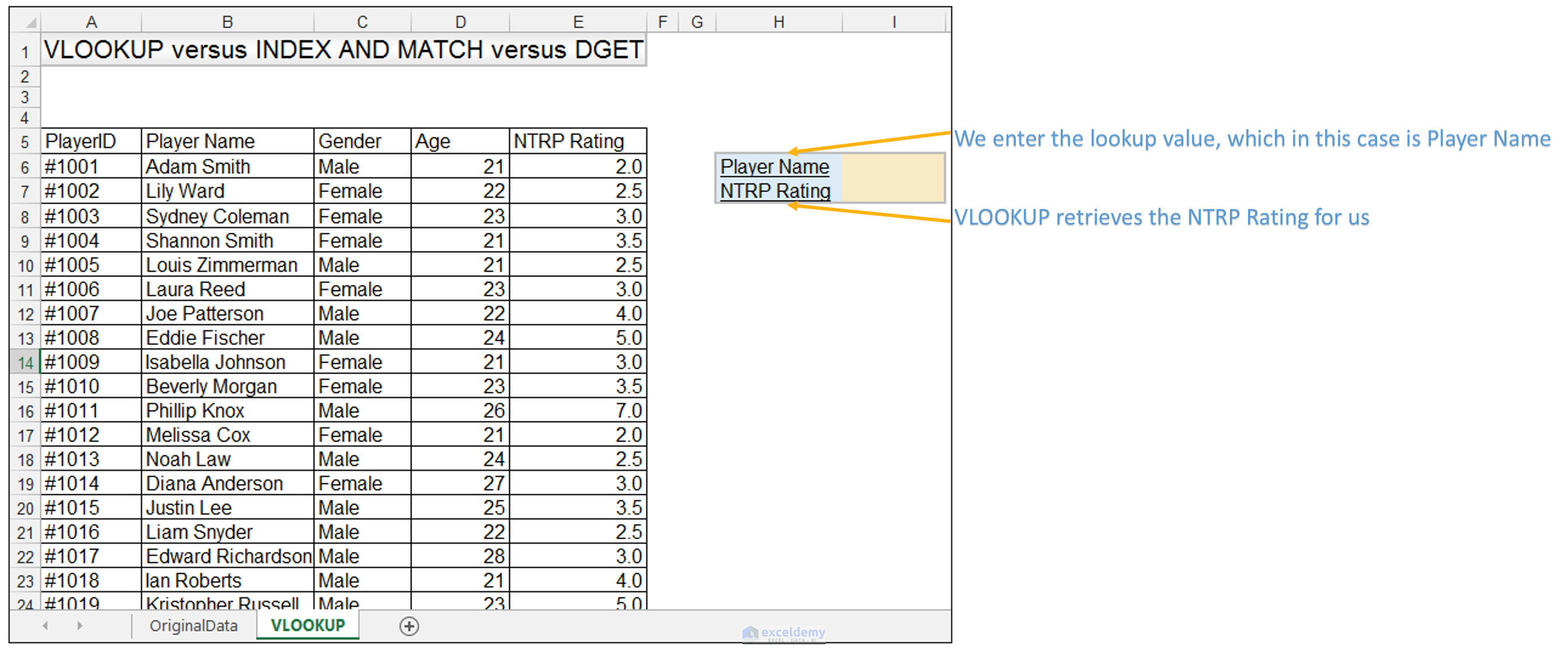2) In Cell I6, we enter the value Ian Roberts, which is the name of the player whose NTRP rating we want to find.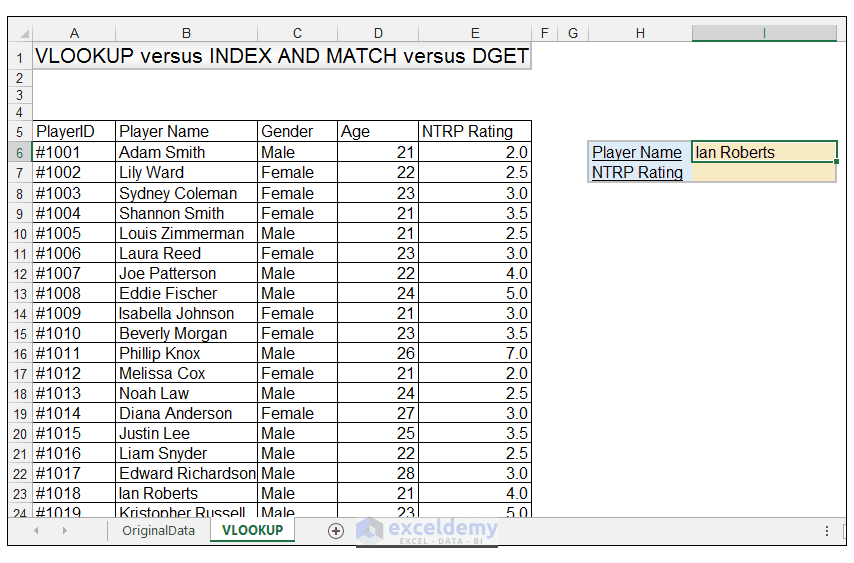3) In Cell I7, we enter the following formula:

=VLOOKUP (I6, \$B\$6: \$E\$105, 4, FALSE)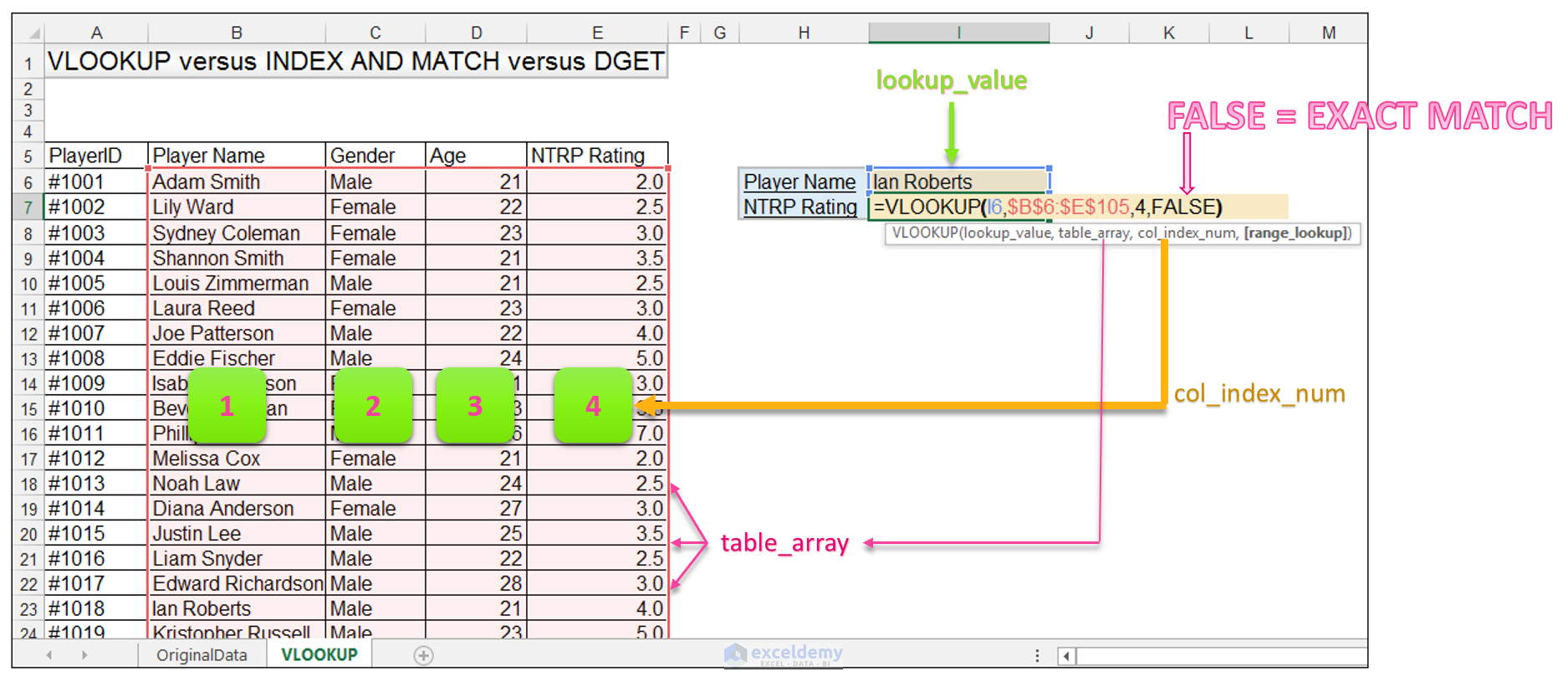4) Upon pressing CTRL-ENTER, VLOOKUP retrieves the value of 4.0 which is the player Ian Roberts’s NTRP rating.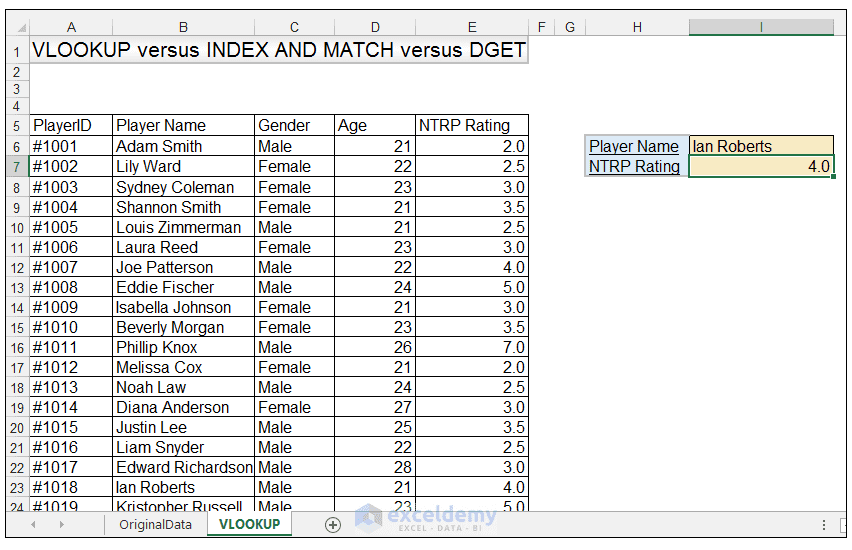The main advantage of the VLOOKUP Function is that it is easy to learn, additionally many Excel users are familiar with the function.  Thus, when sharing sheets or when collaborating with co-workers, everybody is on the same page when it comes to understanding and using the formulas in the workbook.

The disadvantage of VLOOKUP is that it can only do lookups from left to right. In other words, one looks up a value in column, and the match must be in column to a right of that value or else VLOOKUP returns an error message.

Additionally, VLOOKUP can be prone to error in larger spreadsheets. So, in these cases its best to try other lookup functions such as the INDEX and MATCH formula combined and the DGET Function.

Read More: VLOOKUP Function in Excel: Learn with Examples

### INDEX and MATCH

1) For our next example, we still want to enter a player’s name and then find their NTRP rating but this time we are going to use INDEX and MATCH, in order to retrieve the rating of the player at hand.

2) In Cell I6, again we enter the value Ian Roberts, which is the name of the player whose NTRP rating we want to find, as in the example above.

3) In Cell I7, we enter the following formula:

=INDEX (\$E\$6: \$E\$105, MATCH (I6, \$B\$6: \$B\$105, 0))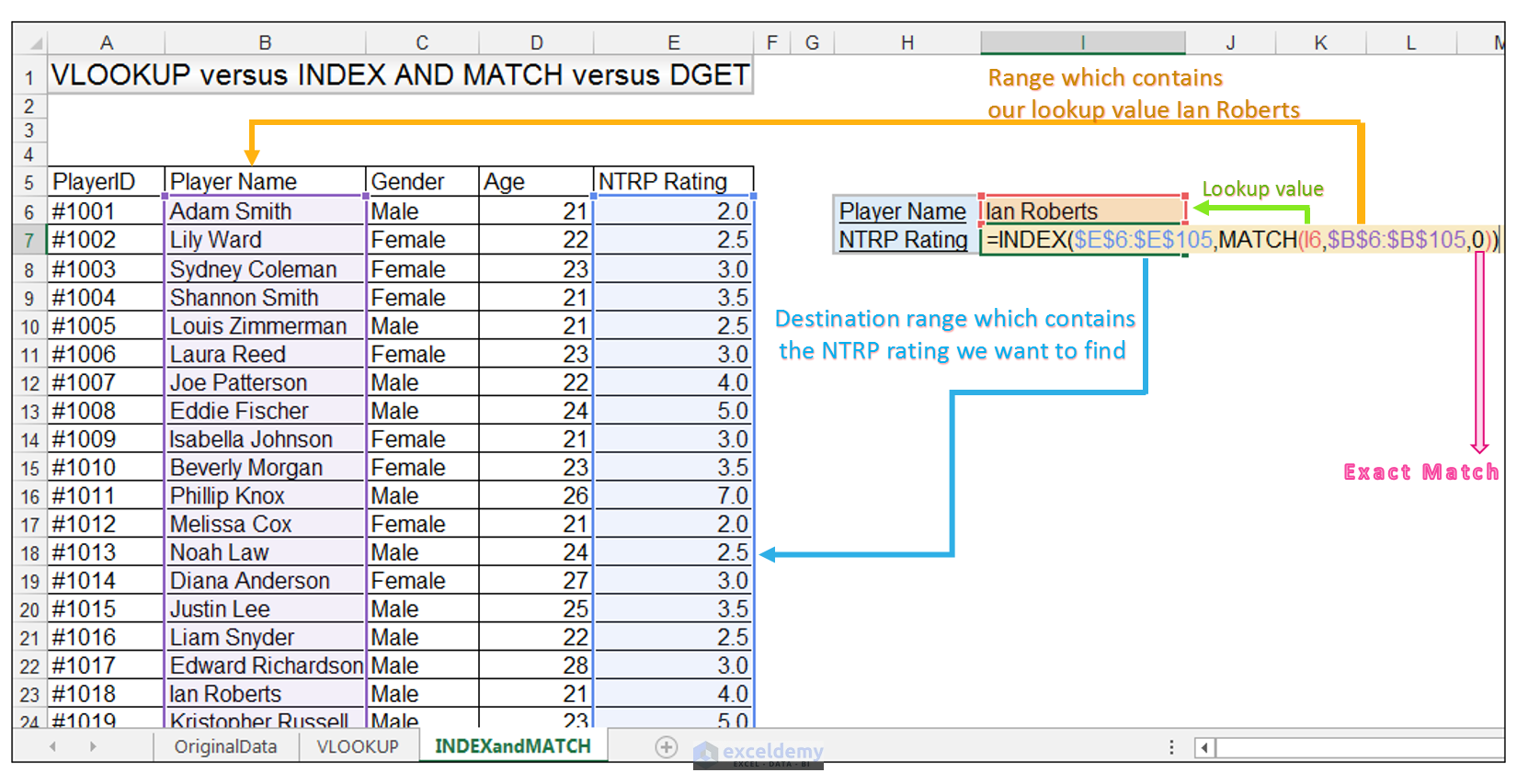4) Upon pressing CTRL-ENTER, the INDEX and MATCH formula retrieves the value of 4.0 which is the player Ian Roberts’s NTRP rating.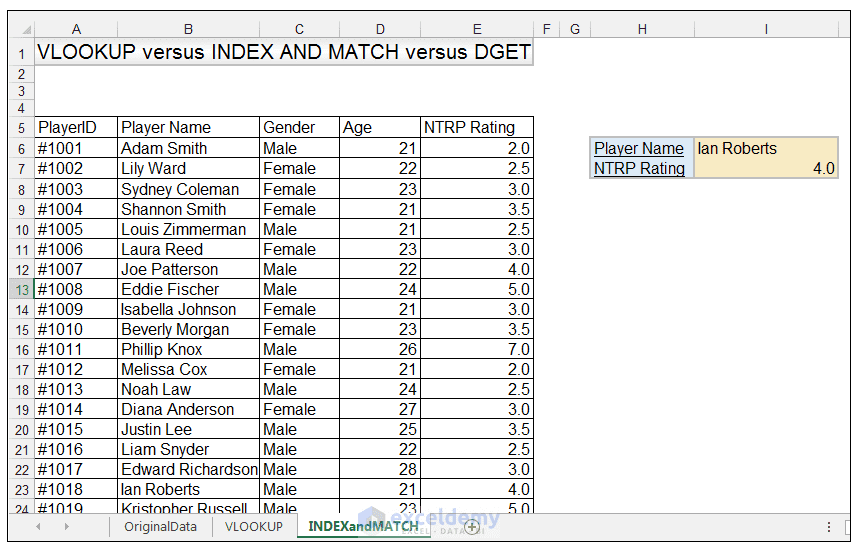The main advantage of using the INDEX and MATCH formula is that it is more versatile than VLOOKUP, and can do left to right and right to left lookups. It is also faster than VLOOKUP in spreadsheets that contain large amounts of data. The main disadvantage is that it is not as straight forward to learn as VLOOKUP and slightly less popular.

Now let’s see how to do the same with the database DGET Function.

## DGET function

1) For the DGET example, we still want to enter a player’s name and then find their rating, however when using the DGET function we have to setup our worksheet a little bit differently. We can use the DGET function to retrieve a single value based on input criteria. So, in this case our input criteria is going to be Ian Roberts, and our field we want to retrieve the matching NTRP Rating from, is NTRP rating.

2) We setup our criteria range in A2:E3 as shown, the field names in the criteria must match the column names of the database range. We enter the value Ian Roberts in Cell B3 as shown below.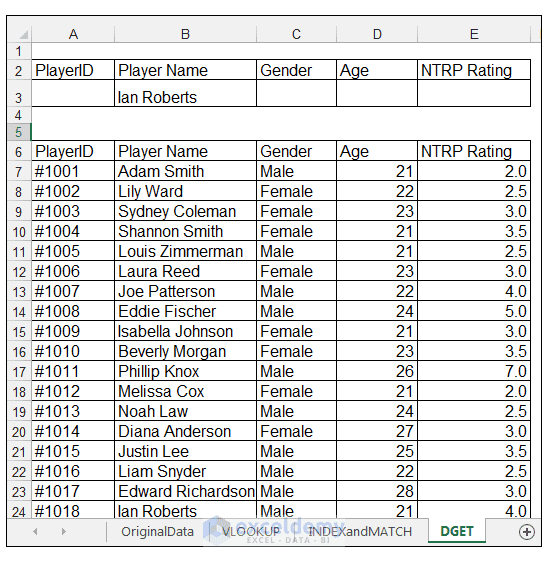3) Now that we have setup our criteria range, in Cell I7 we type the following formula:

=DGET (\$A\$6: \$E\$106, “NTRP Rating”, \$A\$2: \$E\$3)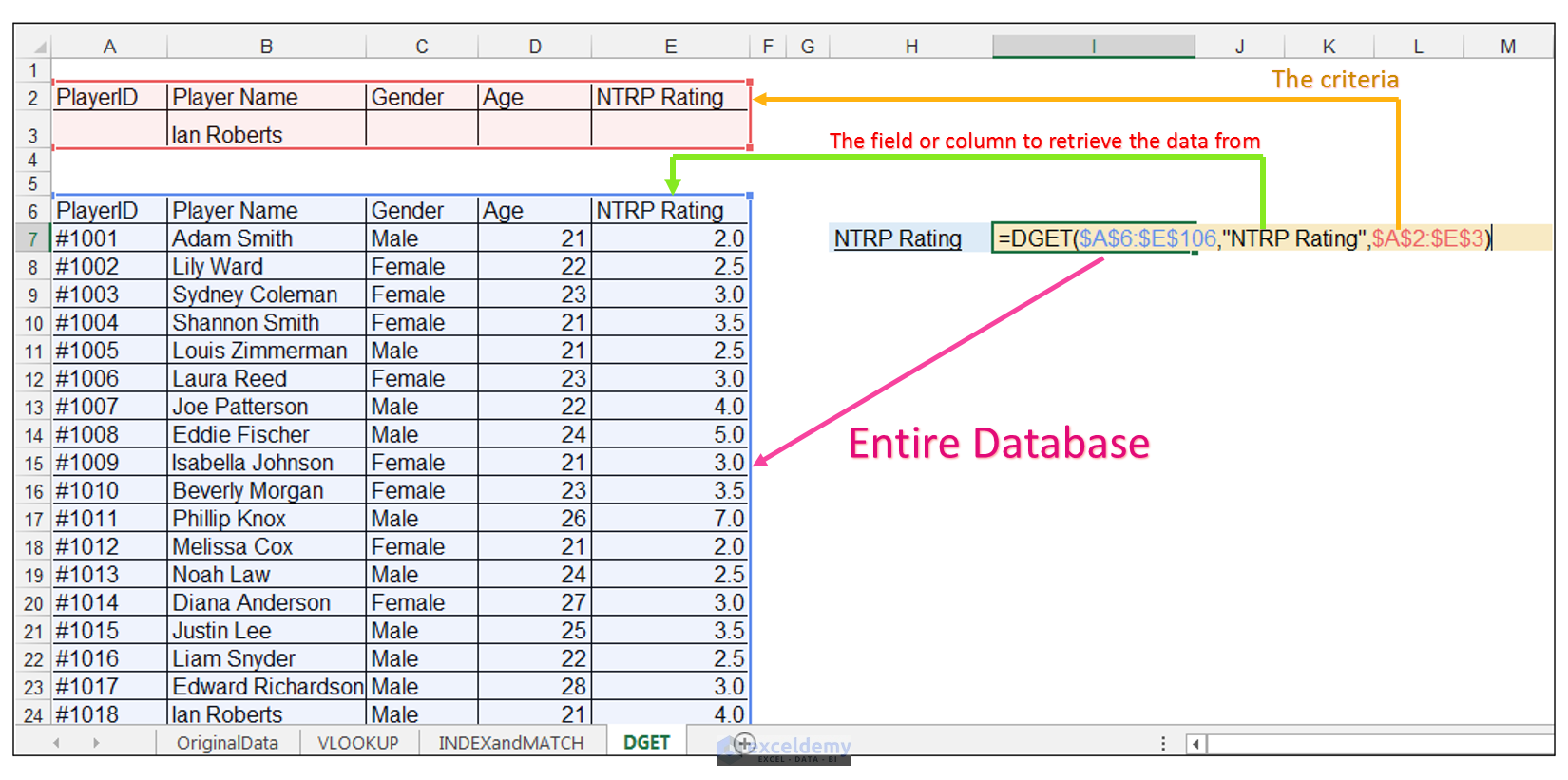4) Upon pressing CTRL-ENTER, DGET retrieves the value of 4.0 which is the player Ian Roberts’s NTRP rating.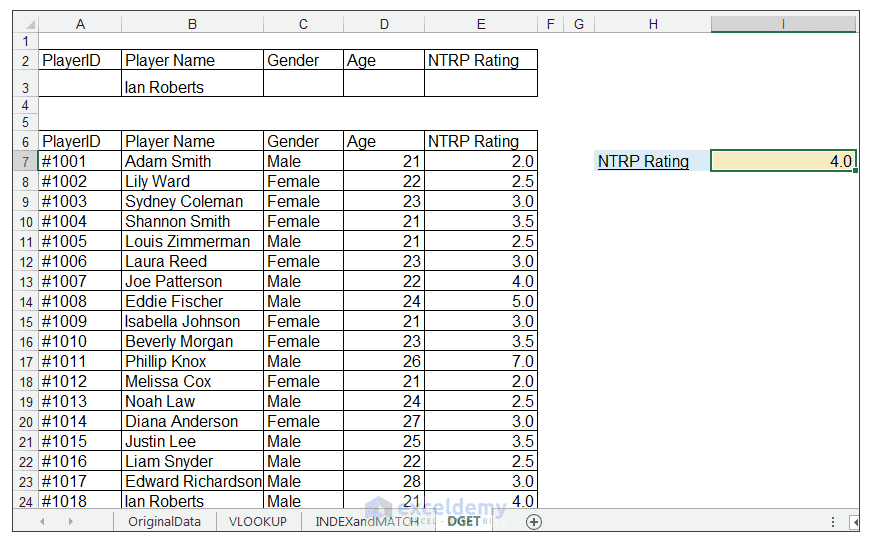The main advantage of using the DGET function is that can handle multiple criteria, and larger data sets with ease. The main disadvantage however, is that it is not as widely utilized as VLOOKUP and INDEX and MATCH, so other users of the spreadsheet would be unfamiliar with it. Also, the setup required initially can prove counter-intuitive to some users, since it has a slight SQL query feel to it.

## Conclusion

Excel has many useful lookup functions, that can assist with retrieving values under specified conditions.

Please feel free to comment and tell us which lookup function you use most often in your spreadsheets.

VLOOKUP-vs-INDEX-MATCH-vs-DGET.xlsx

VLOOKUP Function in Excel: Learn with Examples

How to Use Excel Database Functions DGET, DAVERAGE, & DMAX

Using Excel Worksheet Functions INDEX & MATCH in VBA Code!

Taryn is a Microsoft Certified Professional, who has used Office Applications such as Excel and Access extensively, in her interdisciplinary academic career and work experience. She has a background in biochemistry, Geographical Information Systems (GIS) and biofuels. She enjoys showcasing the functionality of Excel in various disciplines.

1. Replykhiari March 31, 2017 at 1:15 PM

Thank you very much, it’s interinsting

• Reply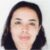March 31, 2017 at 9:56 PM

You are very welcome. Thank you for reading and doing Excel with us 🙂

2. ReplyAnna April 16, 2017 at 7:14 AM

This was well written and easy to understand. I must admit I was not familiar with DGET but I will try using it. Thanks!

• ReplyApril 16, 2017 at 9:37 PM

You are most welcome. I’m glad you found the tutorial useful and yes the database functions are very helpful when it comes to looking up data with criteria 🙂

3. ReplySurya March 28, 2018 at 7:20 AM

Thank you for the easy to understand and useful tutorial. Always look forward to your tutorials posted on this website.

4. ReplyMalcolm B June 3, 2019 at 1:34 AM

Main disadvantage with INDEX/MATCH is that you have to repeat the same range twice which makes maintenance more of an issue (unless you only used named ranges)
Main disadvantage of DGET is that you can’t fill the query down a column to get multiple answers.

• ReplyJune 3, 2019 at 6:09 PM